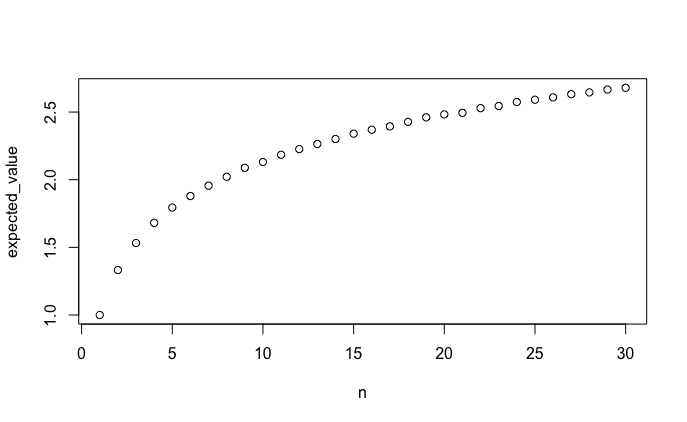# JUNTO Practice: Probability, String Loops

Discussed on June 16, 2020.

Start with n strings, which of course have 2n ends. Then randomly pair the ends and tie together each pair. (Therefore you join each of the n randomly chosen pairs.) Let L be the number of resulting loops. Compute E(L).

## Solutions

Click to see:

### Oscar Martinez

• For n = 1, E(L) = 1.

• When n > 1

• With (2n-1)-1 probability, the problem becomes n-1 plus the 1 loop created by looping on itself.
• With 1 - (2n-1)-1 probability, it is just n-1, as the string becomes part of another string to effectively reduce the number of strings by 1 (hence n-1).
• As a result, E(L) for any n can be represented as:

1 + ... + (2i-1)-1 + ... (2n-1)-1

### John Lekberg

For n strings, I believe that

E(L) ≈ 1 + ½ log(n)

I wrote a Python function that samples L:

``````import csv
import random
import statistics
import sys

# I represent a string end with a tuple of (string, end).
# Originally, I used a namedtuple, but I found that using a
# tuple gave significant speed improvements.

# I represent the "ends" of the string with True and False.
# Originally, I used an Enum, but I found that using
# True/False gave significant speed improvements.

# Overall, the changes I made to optimize the code gave it

def random_number_of_loops(n):
"""Samples the random variable "the number
of resulting loops" for n strings.
"""

remaining = [
(string, end)
for string in range(n)
for end in (True, False)
]

pair = {}
n_loops = 0

random.shuffle(remaining)
# Originally, the loop looked like
#
# while remaining:
#     se1 = remaining.pop()
#     se2 = remaining.pop()
#     ...
#
# But I got a good speedup from using the for-loop and
# indexing.
for i in range(0, 2 * n, 2):
se1 = remaining[i]
se2 = remaining[i + 1]

pair[se1] = se2
pair[se2] = se1

# Originally, this block was a separate function.
# But I got a good speedup from inlining it.
loop = None
se = se1
while True:
se = (se, not se)
if se == se1:
loop = True
break
if se not in pair:
loop = False
break
se = pair[se]
if se == se1:
loop = True
break

if loop:
n_loops += 1

return n_loops
``````

Then, I wrote a Python function to generate a dataset of computed expected values for me to analyze:

``````def generate_data(*, up_to, n_samples):
writer = csv.writer(sys.stdout)
writer.writerow(["n", "expected_value"])
for n in range(1, up_to + 1):
sample = [
random_number_of_loops(n)
for _ in range(n_samples)
]
writer.writerow([n, statistics.mean(sample)])

generate_data(up_to=30, n_samples=50000)
``````
``````n,expected_value
1,1
2,1.33208
3,1.532
4,1.68118
5,1.79434
6,1.87926
7,1.95622
8,2.02166
9,2.0881
10,2.13078
11,2.1843
12,2.22632
13,2.26404
14,2.30072
15,2.34028
16,2.36918
17,2.39412
18,2.4273
19,2.46082
20,2.48276
21,2.49408
22,2.5287
23,2.54484
24,2.57436
25,2.59124
26,2.60868
27,2.63214
28,2.6453
29,2.66644
30,2.67922
``````

Then I loaded this data into R to analyze:

``````plot(df)
``````This looks like it could be a logarithmic relationship (y = log(x)) or a power relationship (y = xz), so I created linear models for both:

``````model_pow <- lm(log(expected_value) ~ log(n), data=df)
model_log <- lm(expected_value ~ log(n), data=df)

summary(model_pow)
``````
``````Call:
lm(formula = log(expected_value) ~ log(n), data = df)

Residuals:
Min        1Q    Median        3Q       Max
-0.120232 -0.016768  0.002125  0.025326  0.038374

Coefficients:
Estimate Std. Error t value Pr(>|t|)
(Intercept) 0.120232   0.018923   6.354  7.1e-07 ***
log(n)      0.264709   0.007208  36.725  < 2e-16 ***
---
Signif. codes:  0 ‘***’ 0.001 ‘**’ 0.01 ‘*’ 0.05 ‘.’ 0.1 ‘ ’ 1

Residual standard error: 0.03301 on 28 degrees of freedom
Multiple R-squared:  0.9797,	Adjusted R-squared:  0.9789
F-statistic:  1349 on 1 and 28 DF,  p-value: < 2.2e-16
``````
``````summary(model_log)
``````
``````Call:
lm(formula = expected_value ~ log(n), data = df)

Residuals:
Min         1Q     Median         3Q        Max
-0.0095502 -0.0024057  0.0003316  0.0029467  0.0076283

Coefficients:
Estimate Std. Error t value Pr(>|t|)
(Intercept) 0.992372   0.002281   435.0   <2e-16 ***
log(n)      0.496386   0.000869   571.2   <2e-16 ***
---
Signif. codes:  0 ‘***’ 0.001 ‘**’ 0.01 ‘*’ 0.05 ‘.’ 0.1 ‘ ’ 1

Residual standard error: 0.00398 on 28 degrees of freedom
Multiple R-squared:  0.9999,	Adjusted R-squared:  0.9999
F-statistic: 3.263e+05 on 1 and 28 DF,  p-value: < 2.2e-16
``````

From this information, I think that `model_log` explains the data better.

• The intercept is 0.992372.
• The coefficient for log n is 0.496386.

As a result, I believe that

E(L) ≈ 1 + ½ log(n)

### Daniel Bassett

``````import sympy as sy

"""Premise 1: For any number of strings 'n', there are two
possibilities on the first draw out of the bag: the same
string is chosen and a loop is created or two different
strings are chosen and tied together. Either case leaves the
bag with n-1 strings. Therefore, the expectation of a loop
on the first pick is E(n), the second E(n) + E(n-1) and so
on for n strings.

Premise 2: What is the probability of making a loop on the
first draw? Consider three strings: there are 6 total ends,
I choose one end, what is the probability of choosing the
other end of the string? There are 5 remaining ends total,
therefore, 1 in 5.

More abstractly, it follows that the probability of a loop
is the total number of strings divided by the total number
of ends times the remaining ends after one is chosen,
divided by 2:

n/(2n(2n-1)/2)
which simplifies to:
1/(2n-1)

Conclusion: We can plug our finding from Premise2 into
Premise1:

1/(2n-1) + 1/(2(n-1)-1) + ... + 1
=1/(2n-1) + 1/(2n-3) + ... + 1

or summation(1/(2i-1), (i, 1, n)

Below is a Python program using sympy that can calculate
E(n) for any given n strings."""

n = int(input("Enter Number of Strings: "))
x = sy.Symbol('x')
expected = sy.summation(1/(2*x-1), (x, 1, n))
print("Expected Loops = ", expected)
``````